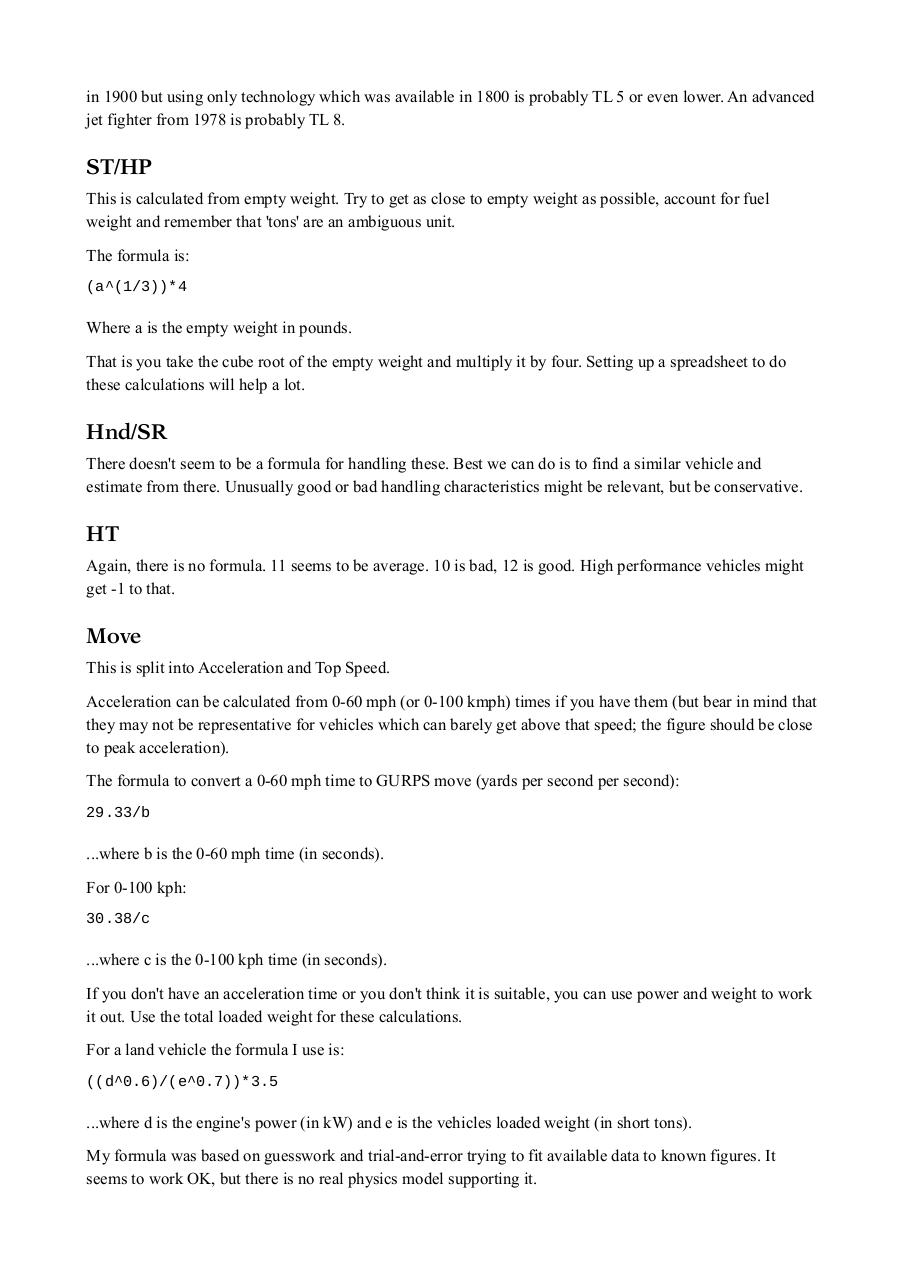# GURPS 4E Vehicles tg Guide.pdfPage 1 2 3 4 5 6 7 8

#### Text preview

in 1900 but using only technology which was available in 1800 is probably TL 5 or even lower. An advanced
jet fighter from 1978 is probably TL 8.

ST/HP
This is calculated from empty weight. Try to get as close to empty weight as possible, account for fuel
weight and remember that 'tons' are an ambiguous unit.
The formula is:
(a^(1/3))*4

Where a is the empty weight in pounds.
That is you take the cube root of the empty weight and multiply it by four. Setting up a spreadsheet to do
these calculations will help a lot.

Hnd/SR
There doesn't seem to be a formula for handling these. Best we can do is to find a similar vehicle and
estimate from there. Unusually good or bad handling characteristics might be relevant, but be conservative.

HT
Again, there is no formula. 11 seems to be average. 10 is bad, 12 is good. High performance vehicles might
get -1 to that.

Move
This is split into Acceleration and Top Speed.
Acceleration can be calculated from 0-60 mph (or 0-100 kmph) times if you have them (but bear in mind that
they may not be representative for vehicles which can barely get above that speed; the figure should be close
to peak acceleration).
The formula to convert a 0-60 mph time to GURPS move (yards per second per second):
29.33/b

...where b is the 0-60 mph time (in seconds).
For 0-100 kph:
30.38/c

...where c is the 0-100 kph time (in seconds).
If you don't have an acceleration time or you don't think it is suitable, you can use power and weight to work
it out. Use the total loaded weight for these calculations.
For a land vehicle the formula I use is:
((d^0.6)/(e^0.7))*3.5

...where d is the engine's power (in kW) and e is the vehicles loaded weight (in short tons).
My formula was based on guesswork and trial-and-error trying to fit available data to known figures. It
seems to work OK, but there is no real physics model supporting it.